GeeksforGeeks App
Open AppBrowser
Continue

# Boruvka’s algorithm | Greedy Algo-9

We have discussed the following topics on Minimum Spanning Tree.
Applications of Minimum Spanning Tree Problem
Kruskal’s Minimum Spanning Tree Algorithm
Prim’s Minimum Spanning Tree Algorithm
In this post, Boruvka’s algorithm is discussed. Like Prim’s and Kruskal’s, Boruvka’s algorithm is also a Greedy algorithm. Below is a complete algorithm.

```1) Input is a connected, weighted and un-directed graph.
2) Initialize all vertices as individual components (or sets).
3) Initialize MST as empty.
4) While there are more than one components, do following
for each component.
a)  Find the closest weight edge that connects this
component to any other component.
5) Return MST.```

Below is the idea behind the above algorithm (The idea is the same as Prim’s MST algorithm).
A spanning tree means all vertices must be connected. So the two disjoint subsets (discussed above) of vertices must be connected to make a Spanning Tree. And they must be connected with the minimum weight edge to make it a Minimum Spanning Tree.
Let us understand the algorithm in the below example.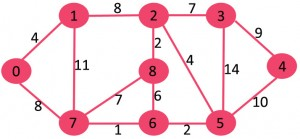Initially, MST is empty. Every vertex is single component as highlighted in blue color in the below diagram.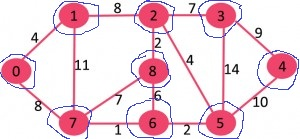For every component, find the cheapest edge that connects it to some other component.

```Component                Cheapest Edge that connects
it to some other component
{0}                           0-1
{1}                           0-1
{2}                           2-8
{3}                           2-3
{4}                           3-4
{5}                           5-6
{6}                           6-7
{7}                           6-7
{8}                           2-8 ```

The cheapest edges are highlighted with green color. Now MST becomes {0-1, 2-8, 2-3, 3-4, 5-6, 6-7}.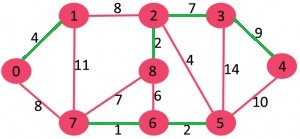After above step, components are {{0,1}, {2,3,4,8}, {5,6,7}}. The components are encircled with blue color.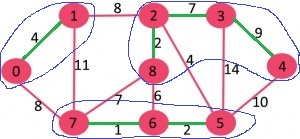We again repeat the step, i.e., for every component, find the cheapest edge that connects it to some other component.

```Component                Cheapest Edge that connects
it to some other component
{0,1}                        1-2 (or 0-7)
{2,3,4,8}                    2-5
{5,6,7}                      2-5```

The cheapest edges are highlighted with green color. Now MST becomes {0-1, 2-8, 2-3, 3-4, 5-6, 6-7, 1-2, 2-5}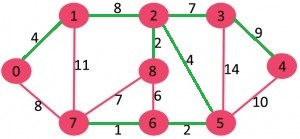At this stage, there is only one component {0, 1, 2, 3, 4, 5, 6, 7, 8} which has all edges. Since there is only one component left, we stop and return MST.

Implementation: Below is the implementation of the above algorithm. The input graph is represented as a collection of edges and union-find data structure is used to keep track of components.

## C++

 `// Boruvka's algorithm to find Minimum Spanning``// Tree of a given connected, undirected and weighted graph``#include ``using` `namespace` `std;` `// Class to represent a graph``class` `Graph {``    ``int` `V; ``// No. of vertices``    ``vector >graph; ``// default dictionary to store graph` `    ``// A utility function to find set of an element i``    ``// (uses path compression technique)``    ``int` `find(vector<``int``>& parent, ``int` `i)``    ``{``        ``if` `(parent[i] == i) {``            ``return` `i;``        ``}``        ``return` `find(parent, parent[i]);``    ``}` `    ``// A function that does union of two sets of x and y``    ``// (uses union by rank)``    ``void` `unionSet(vector<``int``>& parent, vector<``int``>& rank,``                  ``int` `x, ``int` `y)``    ``{``        ``int` `xroot = find(parent, x);``        ``int` `yroot = find(parent, y);` `        ``// Attach smaller rank tree under root of high rank``        ``// tree (Union by Rank)``        ``if` `(rank[xroot] < rank[yroot]) {``            ``parent[xroot] = yroot;``        ``}``        ``else` `if` `(rank[xroot] > rank[yroot]) {``            ``parent[yroot] = xroot;``        ``}``        ``// If ranks are same, then make one as root and``        ``// increment its rank by one``        ``else` `{``            ``parent[yroot] = xroot;``            ``rank[xroot]++;``        ``}``    ``}` `public``:``    ``Graph(``int` `vertices)``    ``{``        ``V = vertices;``        ``graph = vector >();``    ``}` `    ``// function to add an edge to graph``    ``void` `addEdge(``int` `u, ``int` `v, ``int` `w)``    ``{``        ``graph.push_back({ u, v, w });``    ``}` `    ``// The main function to construct MST using Kruskal's``    ``// algorithm``    ``void` `boruvkaMST()``    ``{``        ``vector<``int``> parent(V);` `        ``// An array to store index of the cheapest edge of``        ``// subset. It store [u,v,w] for each component``        ``vector<``int``> rank(V);``        ``vector > cheapest(V,``                                      ``vector<``int``>(3, -1));` `        ``// Initially there are V different trees.``        ``// Finally there will be one tree that will be MST``        ``int` `numTrees = V;``        ``int` `MSTweight = 0;` `        ``// Create V subsets with single elements``        ``for` `(``int` `node = 0; node < V; node++) {``            ``parent[node] = node;``            ``rank[node] = 0;``        ``}` `        ``// Keep combining components (or sets) until all``        ``// components are not combined into single MST``        ``while` `(numTrees > 1) {` `            ``// Traverse through all edges and update``            ``// cheapest of every component``            ``for` `(``int` `i = 0; i < graph.size(); i++) {` `                ``// Find components (or sets) of two corners``                ``// of current edge``                ``int` `u = graph[i], v = graph[i],``                    ``w = graph[i];``                ``int` `set1 = find(parent, u),``                    ``set2 = find(parent, v);` `                ``// If two corners of current edge belong to``                ``// same set, ignore current edge. Else check``                ``// if current edge is closer to previous``                ``// cheapest edges of set1 and set2``                ``if` `(set1 != set2) {``                    ``if` `(cheapest[set1] == -1``                        ``|| cheapest[set1] > w) {``                        ``cheapest[set1] = { u, v, w };``                    ``}``                    ``if` `(cheapest[set2] == -1``                        ``|| cheapest[set2] > w) {``                        ``cheapest[set2] = { u, v, w };``                    ``}``                ``}``            ``}` `            ``// Consider the above picked cheapest edges and``            ``// add them to MST``            ``for` `(``int` `node = 0; node < V; node++) {` `                ``// Check if cheapest for current set exists``                ``if` `(cheapest[node] != -1) {``                    ``int` `u = cheapest[node],``                        ``v = cheapest[node],``                        ``w = cheapest[node];``                    ``int` `set1 = find(parent, u),``                        ``set2 = find(parent, v);``                    ``if` `(set1 != set2) {``                        ``MSTweight += w;``                        ``unionSet(parent, rank, set1, set2);``                        ``printf``(``"Edge %d-%d with weight %d "``                               ``"included in MST\n"``,``                               ``u, v, w);``                        ``numTrees--;``                    ``}``                ``}``            ``}``            ``for` `(``int` `node = 0; node < V; node++) {` `                ``// reset cheapest array``                ``cheapest[node] = -1;``            ``}``        ``}``        ``printf``(``"Weight of MST is %d\n"``, MSTweight);``    ``}``};``int` `main()``{``    ``Graph g(4);``    ``g.addEdge(0, 1, 10);``    ``g.addEdge(0, 2, 6);``    ``g.addEdge(0, 3, 5);``    ``g.addEdge(1, 3, 15);``    ``g.addEdge(2, 3, 4);` `    ``g.boruvkaMST();``}``// This code is contributed by prajwal kandekar`

## Java

 `// Boruvka's algorithm to find Minimum Spanning``// Tree of a given connected, undirected and weighted graph``import` `java.util.*;` `// Class to represent a graph``class` `Graph {``  ``private` `int` `V; ``// No. of vertices``  ``private` `List >``    ``graph; ``// default dictionary to store graph` `  ``Graph(``int` `vertices)``  ``{``    ``V = vertices;``    ``graph = ``new` `ArrayList<>();``  ``}` `  ``// function to add an edge to graph``  ``void` `addEdge(``int` `u, ``int` `v, ``int` `w)``  ``{``    ``graph.add(Arrays.asList(u, v, w));``  ``}` `  ``// A utility function to find set of an element i``  ``// (uses path compression technique)``  ``private` `int` `find(List parent, ``int` `i)``  ``{``    ``if` `(parent.get(i) == i) {``      ``return` `i;``    ``}``    ``return` `find(parent, parent.get(i));``  ``}` `  ``// A function that does union of two sets of x and y``  ``// (uses union by rank)``  ``private` `void` `unionSet(List parent,``                        ``List rank, ``int` `x, ``int` `y)``  ``{``    ``int` `xroot = find(parent, x);``    ``int` `yroot = find(parent, y);` `    ``// Attach smaller rank tree under root of high rank``    ``// tree (Union by Rank)``    ``if` `(rank.get(xroot) < rank.get(yroot)) {``      ``parent.set(xroot, yroot);``    ``}``    ``else` `if` `(rank.get(xroot) > rank.get(yroot)) {``      ``parent.set(yroot, xroot);``    ``}` `    ``// If ranks are same, then make one as root and``    ``// increment its rank by one``    ``else` `{``      ``parent.set(yroot, xroot);``      ``rank.set(xroot, rank.get(xroot) + ``1``);``    ``}``  ``}` `  ``// The main function to construct MST using Kruskal's``  ``// algorithm``  ``void` `boruvkaMST()``  ``{``    ``List parent = ``new` `ArrayList<>();` `    ``// An array to store index of the cheapest edge of``    ``// subset. It store [u,v,w] for each component``    ``List rank = ``new` `ArrayList<>();` `    ``List > cheapest = ``new` `ArrayList<>();` `    ``// Initially there are V different trees.``    ``// Finally there will be one tree that will be MST``    ``int` `numTrees = V;``    ``int` `MSTweight = ``0``;` `    ``// Create V subsets with single elements``    ``for` `(``int` `node = ``0``; node < V; node++) {``      ``parent.add(node);``      ``rank.add(``0``);``      ``cheapest.add(Arrays.asList(-``1``, -``1``, -``1``));``    ``}` `    ``// Keep combining components (or sets) until all``    ``// components are not combined into single MST``    ``while` `(numTrees > ``1``) {` `      ``// Traverse through all edges and update``      ``// cheapest of every component``      ``for` `(List edge : graph) {` `        ``// Find components (or sets) of two corners``        ``// of current edge``        ``int` `u = edge.get(``0``), v = edge.get(``1``),``        ``w = edge.get(``2``);``        ``int` `set1 = find(parent, u),``        ``set2 = find(parent, v);` `        ``// If two corners of current edge belong to``        ``// same set, ignore current edge. Else check``        ``// if current edge is closer to previous``        ``// cheapest edges of set1 and set2``        ``if` `(set1 != set2) {``          ``if` `(cheapest.get(set1).get(``2``) == -``1``              ``|| cheapest.get(set1).get(``2``) > w) {``            ``cheapest.set(``              ``set1, Arrays.asList(u, v, w));``          ``}``          ``if` `(cheapest.get(set2).get(``2``) == -``1``              ``|| cheapest.get(set2).get(``2``) > w) {``            ``cheapest.set(``              ``set2, Arrays.asList(u, v, w));``          ``}``        ``}``      ``}` `      ``// Consider the above picked cheapest edges and``      ``// add them to MST``      ``for` `(``int` `node = ``0``; node < V; node++) {` `        ``// Check if cheapest for current set exists``        ``if` `(cheapest.get(node).get(``2``) != -``1``) {``          ``int` `u = cheapest.get(node).get(``0``),``          ``v = cheapest.get(node).get(``1``),``          ``w = cheapest.get(node).get(``2``);``          ``int` `set1 = find(parent, u),``          ``set2 = find(parent, v);``          ``if` `(set1 != set2) {``            ``MSTweight += w;``            ``unionSet(parent, rank, set1, set2);``            ``System.out.printf(``              ``"Edge %d-%d with weight %d included in MST\n"``,``              ``u, v, w);``            ``numTrees--;``          ``}``        ``}``      ``}``      ``for` `(List list : cheapest) {``        ``// reset cheapest array``        ``list.set(``2``, -``1``);``      ``}``    ``}``    ``System.out.printf(``"Weight of MST is %d\n"``,``                      ``MSTweight);``  ``}``}` `class` `GFG {``  ``public` `static` `void` `main(String[] args)``  ``{``    ``Graph g = ``new` `Graph(``4``);``    ``g.addEdge(``0``, ``1``, ``10``);``    ``g.addEdge(``0``, ``2``, ``6``);``    ``g.addEdge(``0``, ``3``, ``5``);``    ``g.addEdge(``1``, ``3``, ``15``);``    ``g.addEdge(``2``, ``3``, ``4``);``    ``g.boruvkaMST();``  ``}``}``// This code is contributed by prasad264`

## Python

 `# Boruvka's algorithm to find Minimum Spanning``# Tree of a given connected, undirected and weighted graph` `from` `collections ``import` `defaultdict` `#Class to represent a graph``class` `Graph:` `    ``def` `__init__(``self``,vertices):``        ``self``.V``=` `vertices ``#No. of vertices``        ``self``.graph ``=` `[] ``# default dictionary to store graph``        ` ` ` `    ``# function to add an edge to graph``    ``def` `addEdge(``self``,u,v,w):``        ``self``.graph.append([u,v,w])` `    ``# A utility function to find set of an element i``    ``# (uses path compression technique)``    ``def` `find(``self``, parent, i):``        ``if` `parent[i] ``=``=` `i:``            ``return` `i``        ``return` `self``.find(parent, parent[i])` `    ``# A function that does union of two sets of x and y``    ``# (uses union by rank)``    ``def` `union(``self``, parent, rank, x, y):``        ``xroot ``=` `self``.find(parent, x)``        ``yroot ``=` `self``.find(parent, y)` `        ``# Attach smaller rank tree under root of high rank tree``        ``# (Union by Rank)``        ``if` `rank[xroot] < rank[yroot]:``            ``parent[xroot] ``=` `yroot``        ``elif` `rank[xroot] > rank[yroot]:``            ``parent[yroot] ``=` `xroot``        ``#If ranks are same, then make one as root and increment``        ``# its rank by one``        ``else` `:``            ``parent[yroot] ``=` `xroot``            ``rank[xroot] ``+``=` `1` `    ``# The main function to construct MST using Kruskal's algorithm``    ``def` `boruvkaMST(``self``):``        ``parent ``=` `[]; rank ``=` `[];` `        ``# An array to store index of the cheapest edge of``        ``# subset. It store [u,v,w] for each component``        ``cheapest ``=``[]` `        ``# Initially there are V different trees.``        ``# Finally there will be one tree that will be MST``        ``numTrees ``=` `self``.V``        ``MSTweight ``=` `0` `        ``# Create V subsets with single elements``        ``for` `node ``in` `range``(``self``.V):``            ``parent.append(node)``            ``rank.append(``0``)``            ``cheapest ``=``[``-``1``] ``*` `self``.V``    ` `        ``# Keep combining components (or sets) until all``        ``# components are not combined into single MST` `        ``while` `numTrees > ``1``:` `            ``# Traverse through all edges and update``               ``# cheapest of every component``            ``for` `i ``in` `range``(``len``(``self``.graph)):` `                ``# Find components (or sets) of two corners``                ``# of current edge``                ``u,v,w ``=`  `self``.graph[i]``                ``set1 ``=` `self``.find(parent, u)``                ``set2 ``=` `self``.find(parent ,v)` `                ``# If two corners of current edge belong to``                ``# same set, ignore current edge. Else check if``                ``# current edge is closer to previous``                ``# cheapest edges of set1 and set2``                ``if` `set1 !``=` `set2:    ``                    ` `                    ``if` `cheapest[set1] ``=``=` `-``1` `or` `cheapest[set1][``2``] > w :``                        ``cheapest[set1] ``=` `[u,v,w]` `                    ``if` `cheapest[set2] ``=``=` `-``1` `or` `cheapest[set2][``2``] > w :``                        ``cheapest[set2] ``=` `[u,v,w]` `            ``# Consider the above picked cheapest edges and add them``            ``# to MST``            ``for` `node ``in` `range``(``self``.V):` `                ``#Check if cheapest for current set exists``                ``if` `cheapest[node] !``=` `-``1``:``                    ``u,v,w ``=` `cheapest[node]``                    ``set1 ``=` `self``.find(parent, u)``                    ``set2 ``=` `self``.find(parent ,v)` `                    ``if` `set1 !``=` `set2 :``                        ``MSTweight ``+``=` `w``                        ``self``.union(parent, rank, set1, set2)``                        ``print` `(``"Edge %d-%d with weight %d included in MST"` `%` `(u,v,w))``                        ``numTrees ``=` `numTrees ``-` `1``            ` `            ``#reset cheapest array``            ``cheapest ``=``[``-``1``] ``*` `self``.V` `            ` `        ``print` `(``"Weight of MST is %d"` `%` `MSTweight)``                          `  `    ` `g ``=` `Graph(``4``)``g.addEdge(``0``, ``1``, ``10``)``g.addEdge(``0``, ``2``, ``6``)``g.addEdge(``0``, ``3``, ``5``)``g.addEdge(``1``, ``3``, ``15``)``g.addEdge(``2``, ``3``, ``4``)` `g.boruvkaMST()` `#This code is contributed by Neelam Yadav`

## C#

 `// Boruvka's algorithm to find Minimum Spanning``// Tree of a given connected, undirected and weighted graph``using` `System;``using` `System.Collections.Generic;` `// Class to represent a graph``class` `Graph {``    ``private` `int` `V; ``// No. of vertices``    ``private` `List >``        ``graph; ``// default dictionary to store graph` `    ``// A utility function to find set of an element i``    ``// (uses path compression technique)``    ``private` `int` `Find(List<``int``> parent, ``int` `i)``    ``{``        ``if` `(parent[i] == i) {``            ``return` `i;``        ``}``        ``return` `Find(parent, parent[i]);``    ``}` `    ``// A function that does union of two sets of x and y``    ``// (uses union by rank)``    ``private` `void` `UnionSet(List<``int``> parent, List<``int``> rank,``                          ``int` `x, ``int` `y)``    ``{``        ``int` `xroot = Find(parent, x);``        ``int` `yroot = Find(parent, y);` `        ``// Attach smaller rank tree under root of high rank``        ``// tree (Union by Rank)``        ``if` `(rank[xroot] < rank[yroot]) {``            ``parent[xroot] = yroot;``        ``}``        ``else` `if` `(rank[xroot] > rank[yroot]) {``            ``parent[yroot] = xroot;``        ``}` `        ``// If ranks are same, then make one as root and``        ``// increment its rank by one``        ``else` `{``            ``parent[yroot] = xroot;``            ``rank[xroot]++;``        ``}``    ``}` `    ``public` `Graph(``int` `vertices)``    ``{``        ``V = vertices;``        ``graph = ``new` `List >();``    ``}` `    ``// function to add an edge to graph``    ``public` `void` `AddEdge(``int` `u, ``int` `v, ``int` `w)``    ``{``        ``graph.Add(``new` `List<``int``>{ u, v, w });``    ``}` `    ``// The main function to construct MST using Kruskal's``    ``// algorithm``    ``public` `void` `boruvkaMST()``    ``{``        ``List<``int``> parent = ``new` `List<``int``>();` `        ``// An array to store index of the cheapest edge of``        ``// subset. It store [u,v,w] for each component``        ``List<``int``> rank = ``new` `List<``int``>();``        ``List > cheapest = ``new` `List >();` `        ``// Initially there are V different trees.``        ``// Finally there will be one tree that will be MST``        ``int` `numTrees = V;``        ``int` `MSTweight = 0;` `        ``// Create V subsets with single elements``        ``for` `(``int` `node = 0; node < V; node++) {``            ``parent.Add(node);``            ``rank.Add(0);``            ``cheapest.Add(``new` `List<``int``>{ -1, -1, -1 });``        ``}` `        ``// Keep combining components (or sets) until all``        ``// components are not combined into single MST``        ``while` `(numTrees > 1) {` `            ``// Traverse through all edges and update``            ``// cheapest of every component``            ``for` `(``int` `i = 0; i < graph.Count; i++) {` `                ``// Find components (or sets) of two corners``                ``// of current edge``                ``int` `u = graph[i], v = graph[i],``                    ``w = graph[i];``                ``int` `set1 = Find(parent, u),``                    ``set2 = Find(parent, v);` `                ``// If two corners of current edge belong to``                ``// same set, ignore current edge. Else check``                ``// if current edge is closer to previous``                ``// cheapest edges of set1 and set2``                ``if` `(set1 != set2) {``                    ``if` `(cheapest[set1] == -1``                        ``|| cheapest[set1] > w) {``                        ``cheapest[set1]``                            ``= ``new` `List<``int``>{ u, v, w };``                    ``}``                    ``if` `(cheapest[set2] == -1``                        ``|| cheapest[set2] > w) {``                        ``cheapest[set2]``                            ``= ``new` `List<``int``>{ u, v, w };``                    ``}``                ``}``            ``}` `            ``// Consider the above picked cheapest edges and``            ``// add them to MST``            ``for` `(``int` `node = 0; node < V; node++) {` `                ``// Check if cheapest for current set exists``                ``if` `(cheapest[node] != -1) {``                    ``int` `u = cheapest[node],``                        ``v = cheapest[node],``                        ``w = cheapest[node];``                    ``int` `set1 = Find(parent, u),``                        ``set2 = Find(parent, v);``                    ``if` `(set1 != set2) {``                        ``MSTweight += w;``                        ``UnionSet(parent, rank, set1, set2);``                        ``Console.WriteLine(``                            ``"Edge {0}-{1} with weight {2} included in MST"``,``                            ``u, v, w);``                        ``numTrees--;``                    ``}``                ``}``            ``}``            ``for` `(``int` `node = 0; node < V; node++) {``                ``// reset cheapest array``                ``cheapest[node] = -1;``            ``}``        ``}``        ``Console.WriteLine(``"Weight of MST is {0}"``,``                          ``MSTweight);``    ``}``}` `public` `class` `GFG {``    ``static` `void` `Main(``string``[] args)``    ``{``        ``Graph g = ``new` `Graph(4);``        ``g.AddEdge(0, 1, 10);``        ``g.AddEdge(0, 2, 6);``        ``g.AddEdge(0, 3, 5);``        ``g.AddEdge(1, 3, 15);``        ``g.AddEdge(2, 3, 4);``        ``g.boruvkaMST();``    ``}``}``// This code is contributed by prasad264`

## Javascript

 `// Boruvka's algorithm to find Minimum Spanning``// Tree of a given connected, undirected and weighted graph` `// Class to represent a graph``class Graph {``    ``constructor(vertices) {``        ``this``.V = vertices; ``// No. of vertices``        ``this``.graph = []; ``// default dictionary to store graph``    ``}` `    ``// function to add an edge to graph``    ``addEdge(u, v, w) {``        ``this``.graph.push([u, v, w]);``    ``}` `    ``// A utility function to find set of an element i``    ``// (uses path compression technique)``    ``find(parent, i) {``        ``if` `(parent[i] === i) {``            ``return` `i;``        ``}``        ``return` `this``.find(parent, parent[i]);``    ``}``    ` `    ``// A function that does union of two sets of x and y``    ``// (uses union by rank)``    ``union(parent, rank, x, y) {``        ``const xroot = ``this``.find(parent, x);``        ``const yroot = ``this``.find(parent, y);` `        ``// Attach smaller rank tree under root of high rank tree``        ``// (Union by Rank)``        ``if` `(rank[xroot] < rank[yroot]) {``            ``parent[xroot] = yroot;``        ``} ``else` `if` `(rank[xroot] > rank[yroot]) {``            ``parent[yroot] = xroot;``        ``}``        ` `        ``// If ranks are same, then make one as root and increment``        ``// its rank by one``        ``else` `{``            ``parent[yroot] = xroot;``            ``rank[xroot] += 1;``        ``}``    ``}` `    ``//The main function to construct MST using Kruskal's algorithm``    ``boruvkaMST() {``        ``const parent = [];``        ` `        ``// An array to store index of the cheapest edge of``        ``// subset. It store [u,v,w] for each component``        ``const rank = [];``        ``const cheapest = [];` `        ``// Initially there are V different trees.``        ``// Finally there will be one tree that will be MST``        ``let numTrees = ``this``.V;``        ``let MSTweight = 0;` `        ``// Create V subsets with single elements``        ``for` `(let node = 0; node < ``this``.V; node++) {``            ``parent.push(node);``            ``rank.push(0);``            ``cheapest[node] = -1;``        ``}` `        ``// Keep combining components (or sets) until all``        ``// components are not combined into single MST``        ``while` `(numTrees > 1) {``        ` `            ``// Traverse through all edges and update``            ``// cheapest of every component``            ``for` `(let i = 0; i < ``this``.graph.length; i++) {``            ` `                ``// Find components (or sets) of two corners``                ``// of current edge``                ``const [u, v, w] = ``this``.graph[i];``                ``const set1 = ``this``.find(parent, u);``                ``const set2 = ``this``.find(parent, v);` `                ``// If two corners of current edge belong to``                ``// same set, ignore current edge. Else check if``                ``// current edge is closer to previous``                ``// cheapest edges of set1 and set2``                ``if` `(set1 !== set2) {``                    ``if` `(cheapest[set1] === -1 || cheapest[set1] > w) {``                        ``cheapest[set1] = [u, v, w];``                    ``}` `                    ``if` `(cheapest[set2] === -1 || cheapest[set2] > w) {``                        ``cheapest[set2] = [u, v, w];``                    ``}``                ``}``            ``}` `            ``// Consider the above picked cheapest edges and add them``            ``// to MST``            ``for` `(let node = 0; node < ``this``.V; node++) {``            ` `                ``// Check if cheapest for current set exists``                ``if` `(cheapest[node] !== -1) {``                    ``const [u, v, w] = cheapest[node];``                    ``const set1 = ``this``.find(parent, u);``                    ``const set2 = ``this``.find(parent, v);` `                    ``if` `(set1 !== set2) {``                        ``MSTweight += w;``                        ``this``.union(parent, rank, set1, set2);``                        ``console.log(`Edge \${u}-\${v} ``with` `weight \${w} included ``in` `MST`);``                        ``numTrees--;``                    ``}``                ``}``            ``}` `            ``for` `(let node = 0; node < ``this``.V; node++) {``            ` `                ``// reset cheapest array``                ``cheapest[node] = -1;``            ``}``        ``}` `        ``console.log(`Weight of MST is \${MSTweight}`);``    ``}``}` `let g = ``new` `Graph(4);``g.addEdge(0, 1, 10);``g.addEdge(0, 2, 6);``g.addEdge(0, 3, 5);``g.addEdge(1, 3, 15);``g.addEdge(2, 3, 4);` `g.boruvkaMST();` `// This code is contributed by prajwal kandekar`

Output

```Edge 0-3 with weight 5 included in MST
Edge 0-1 with weight 10 included in MST
Edge 2-3 with weight 4 included in MST
Weight of MST is 19```